Shortcuts

# Kendall Rank Corr. Coef.¶

## Module Interface¶

class torchmetrics.KendallRankCorrCoef(variant='b', t_test=False, alternative='two-sided', num_outputs=1, **kwargs)[source]

Computes Kendall Rank Correlation Coefficient: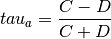where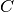represents concordant pairs,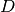stands for discordant pairs.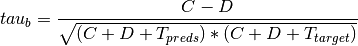whererepresents concordant pairs,stands for discordant pairs and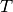represents a total number of ties.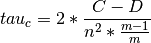whererepresents concordant pairs,stands for discordant pairs,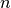is a total number of observations and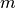is a min of unique values in preds and target sequence.

Definitions according to Definition according to The Treatment of Ties in Ranking Problems.

As input to forward and update the metric accepts the following input:

• preds (Tensor): Sequence of data in float tensor of either shape (N,) or (N,d)

• target (Tensor): Sequence of data in float tensor of either shape (N,) or (N,d)

As output of forward and compute the metric returns the following output:

• kendall (Tensor): A tensor with the correlation tau statistic, and if it is not None, the p-value of corresponding statistical test.

Parameters
Raises
• ValueError – If t_test is not of a type bool

• ValueError – If t_test=True and alternative=None

Example (single output regression):
>>> import torch
>>> from torchmetrics.regression import KendallRankCorrCoef
>>> preds = torch.tensor([2.5, 0.0, 2, 8])
>>> target = torch.tensor([3, -0.5, 2, 1])
>>> kendall = KendallRankCorrCoef()
>>> kendall(preds, target)
tensor(0.3333)

Example (multi output regression):
>>> import torch
>>> from torchmetrics.regression import KendallRankCorrCoef
>>> preds = torch.tensor([[2.5, 0.0], [2, 8]])
>>> target = torch.tensor([[3, -0.5], [2, 1]])
>>> kendall = KendallRankCorrCoef(num_outputs=2)
>>> kendall(preds, target)
tensor([1., 1.])

Example (single output regression with t-test):
>>> import torch
>>> from torchmetrics.regression import KendallRankCorrCoef
>>> preds = torch.tensor([2.5, 0.0, 2, 8])
>>> target = torch.tensor([3, -0.5, 2, 1])
>>> kendall = KendallRankCorrCoef(t_test=True, alternative='two-sided')
>>> kendall(preds, target)
(tensor(0.3333), tensor(0.4969))

Example (multi output regression with t-test):
>>> import torch
>>> from torchmetrics.regression import KendallRankCorrCoef
>>> preds = torch.tensor([[2.5, 0.0], [2, 8]])
>>> target = torch.tensor([[3, -0.5], [2, 1]])
>>> kendall = KendallRankCorrCoef(t_test=True, alternative='two-sided', num_outputs=2)
>>> kendall(preds, target)
(tensor([1., 1.]), tensor([nan, nan]))


Initializes internal Module state, shared by both nn.Module and ScriptModule.

## Functional Interface¶

torchmetrics.functional.kendall_rank_corrcoef(preds, target, variant='b', t_test=False, alternative='two-sided')[source]

Computes Kendall Rank Correlation Coefficient.whererepresents concordant pairs,stands for discordant pairs.whererepresents concordant pairs,stands for discordant pairs andrepresents a total number of ties.whererepresents concordant pairs,stands for discordant pairs,is a total number of observations andis a min of unique values in preds and target sequence.

Definitions according to Definition according to The Treatment of Ties in Ranking Problems.

Parameters
• preds (Tensor) – Sequence of data of either shape (N,) or (N,d)

• target (Tensor) – Sequence of data of either shape (N,) or (N,d)

• variant (Literal[‘a’, ‘b’, ‘c’]) – Indication of which variant of Kendall’s tau to be used

• t_test (bool) – Indication whether to run t-test

• alternative (Optional[Literal[‘two-sided’, ‘less’, ‘greater’]]) – Alternative hypothesis for t-test. Possible values: - ‘two-sided’: the rank correlation is nonzero - ‘less’: the rank correlation is negative (less than zero) - ‘greater’: the rank correlation is positive (greater than zero)

Return type
Returns

Correlation tau statistic (Optional) p-value of corresponding statistical test (asymptotic)

Raises
• ValueError – If t_test is not of a type bool

• ValueError – If t_test=True and alternative=None

Example (single output regression):
>>> from torchmetrics.functional.regression import kendall_rank_corrcoef
>>> preds = torch.tensor([2.5, 0.0, 2, 8])
>>> target = torch.tensor([3, -0.5, 2, 1])
>>> kendall_rank_corrcoef(preds, target)
tensor(0.3333)

Example (multi output regression):
>>> from torchmetrics.functional.regression import kendall_rank_corrcoef
>>> preds = torch.tensor([[2.5, 0.0], [2, 8]])
>>> target = torch.tensor([[3, -0.5], [2, 1]])
>>> kendall_rank_corrcoef(preds, target)
tensor([1., 1.])

Example (single output regression with t-test)
>>> from torchmetrics.functional.regression import kendall_rank_corrcoef
>>> preds = torch.tensor([2.5, 0.0, 2, 8])
>>> target = torch.tensor([3, -0.5, 2, 1])
>>> kendall_rank_corrcoef(preds, target, t_test=True, alternative='two-sided')
(tensor(0.3333), tensor(0.4969))

Example (multi output regression with t-test):
>>> from torchmetrics.functional.regression import kendall_rank_corrcoef
>>> preds = torch.tensor([[2.5, 0.0], [2, 8]])
>>> target = torch.tensor([[3, -0.5], [2, 1]])
>>> kendall_rank_corrcoef(preds, target, t_test=True, alternative='two-sided')
(tensor([1., 1.]), tensor([nan, nan]))


© Copyright Copyright (c) 2020-2023, Lightning-AI et al... Revision 825d17f3.

Built with Sphinx using a theme provided by Read the Docs.
Versions
latest
stable
v0.11.3
v0.11.2
v0.11.1
v0.11.0
v0.10.3
v0.10.2
v0.10.1
v0.10.0
v0.9.3
v0.9.2
v0.9.1
v0.9.0
v0.8.2
v0.8.1
v0.8.0
v0.7.3
v0.7.2
v0.7.1
v0.7.0
v0.6.2
v0.6.1
v0.6.0
v0.5.1
v0.5.0
v0.4.1
v0.4.0
v0.3.2
v0.3.1
v0.3.0
v0.2.0
v0.1.0